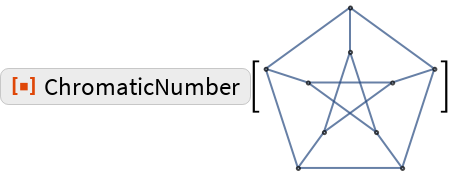#Function Repository Resource:

# ChromaticNumber

Compute the vertex chromatic number of a graph

Contributed by: Szabolcs Horvát and Michael Sollami
 ResourceFunction["ChromaticNumber"][g] computes χ(g) the vertex chromatic number of simple graph g.

## Details and Options

ResourceFunction["ChromaticNumber"] works on both connected and unconnected simple graphs, i.e. undirected graphs containing no self-loops or multiedges.
The chromatic number of a graph is the smallest number of colors needed to color the vertices so that no two adjacent vertices share the same color.
This function uses a linear programming based algorithm, it works well in general but if you need faster performance check out IGChromaticNumber and IGMinimumVertexColoring from the igraph interface IGraph/M.

## Examples

### Basic Examples

Compute chromatic numbers of simple graphs:

 In:=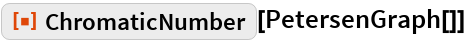Out=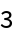Compute chromatic numbers of graphs:

 In:=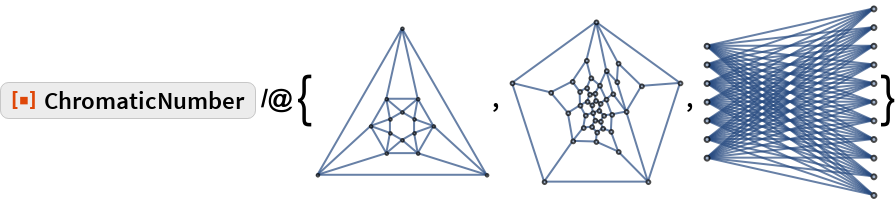Out=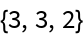Compute the vertex chromatic number of famous graphs:

 In:=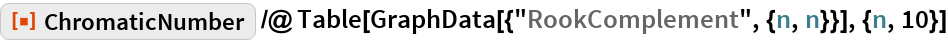Out=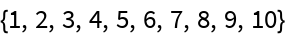### Scope

Works on graphs in either 2D or 3D form:

 In:=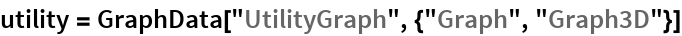Out=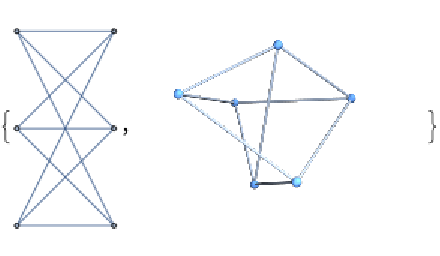In:=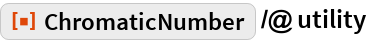Out=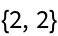Special and corner cases are handled efficiently:

 In:=Out=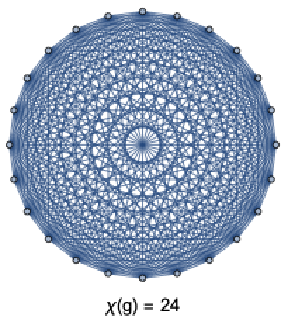### Applications

Compute on larger graphs than was possible before (with Combinatorica`):

 In:=Out=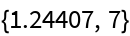### Possible Issues

ChromaticNumber does not work on the output of GraphPlot:

 In:=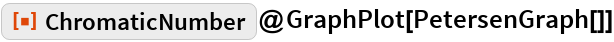Out=